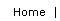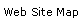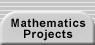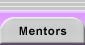# Parity Arguments

If two integers are either both even or both odd, they are said to have the same parity; otherwise they have different parity. Determining the parity of two quantities is often a simple and useful way to prove that the quantities can never be equal. That result, in turn, can be used to demonstrate that a particular situation is impossible.

Parity is just a special case of divisibility. Although we do not have special words for “divisible by 5” or “leaves a remainder when divided by 7,” issues of divisibility arise frequently. For example, a fourth grader was investigating which n by m rectangular regions could be tiled by the pentomino below. The 4 by 10 rectangle shown is once such possibility.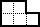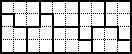A pentomino and a 4 by 10 rectangular tiling

The student conjectured that at least one of the dimensions of the rectangle had to be divisible by 5 for a tiling to be possible. She explained that the area of the pentomino had to divide evenly into the area of the rectangle. Since 5 is prime, the only way the rectangle’s area, mn, could be divisible by 5 was for either m or n to be so. (You can prove an additional condition if you color the rectangle with a checkerboard pattern and think about the parity of the colors covered by each tile).

For practice with proofs that involve parity arguments, see Parity Problems and Parity Problems 2. For practice with, and further information about, divisibility, see Divisibility and Modular Arithmetic.Translations of mathematical formulas for web display were created by tex4ht. © Copyright 2003 Education Development Center, Inc. (EDC)